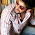## Friday, December 11, 2015

### Sum and Product of Factors, Odd and Even Factors with their Sum, General and Coprime Factor Pairs, Highest Power of Prime Number in a Factorial Video

In this video I have started from where I left in the explanation of Number System. Previously I have uploaded around 21 videos of Number System and now this one is the 22nd second in the row. In this video I have explained how to find out the sum and product of factors of a natural number. The formula and its proof has been covered by me in this video. Formula of sum and product is a bit confusing and it is better to have a look at a proof of it as the proof will help you to remember the formula forever. The sum of factors is basically the product of sum of different geometric progressions formed for different unique prime factors. The product of all factors comes out as the product of all Factor Pairs. Then by the same concept I have explained how by manipulating the powers of different unique prime numbers I have shown how to calculate the number of odd and even factors of a numbers and also how their sum is calculated. Also I have shown how to calculate the number of factors divisible by a particular number say 15 or 20 and how to find out the sum of all the factors divisible by 15 or 20. This is a bit tough concept but once you understand how it is happening it would become very easy for you to solve.Then while solving it I have explained what Factor Pair means. Factor Pairs are those two numbers which when multiplied with each other form the original number. Then I have told how to calculate how we can express a number as a product of any two factors and then how to express it in terms of factor pair which has both terms as coprime. Then I have explained how to find out the highest power of a prime number in a factorial. The logic how this formula will be extended to find out the highest power of a number in a factorial has also been detailed. Then I have solved the below discussed questions in this video. Please free feel to comment and clarify your doubts by using the comment form below. Positive and Negative comments both are welcome.

Question 1.The factorised form of a number N = 24 X 34 X 76. In how many ways can the number N be written as a product of

1.Two Factors ?
2. Two different Factors ?

Question 2. How many factors of 10100 are not an integral power of 1010 ??

Question 3. If N = 23 x 32, then how many sets of two distinct factors of N are coprime to each other ?

Question 4. What is the largest power of 12 that would divide 49! ??

Question 5. If 2100! = (504)p x q, where q is not a multiple of 7, then find a value of p ?

1.sir pls upload video for probability.

2.Sir, I have completed watching all of your number system videos, which you have uploaded in English. But in this video i couldn't understand your language, Please Kindly upload in English. So that every Indian Could understand and make use of your valuable lectures.

3.sir, please explain the derivation of the formula 2^n-1, otherwise the concept of it is remaining hazy. also please explain the derivation of the formula for finding the number of coprime factors of a given number and the highest power of a prime number in a factorial.I would request you to upload videos for these.Thanking you in anticipation...

4.Sir, please upload videos explaining the concept of the divisibility rule of 7, 11 and 13 separately. The combined one that u have uploaded has been of immense help. Thank u!!

5.sir please upload lectures on probability and pnc.I m totally dependent on ur video lectures.

6.sir please upload lectures on probability and pnc.I m totally dependent on ur video lectures.

7.sir are you all ok.....we are concern about health....

8.sir,sorry to say that i did not understand this class...is this topic much more important for ssc cgl

Add a Comment or Query